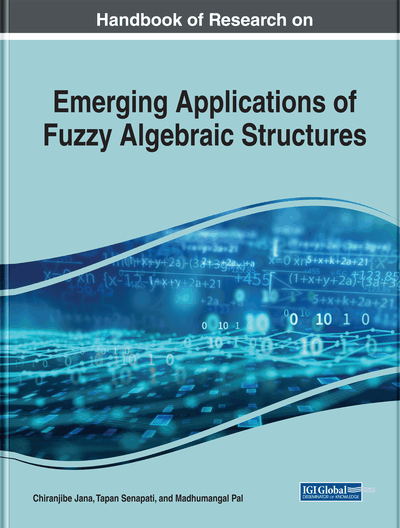# Novel Fuzzy Preview Control With Granular Computing for Underactuated Systems: Underactuated System Case Studies

Srikanth Kavirayani (Gayatri Vidya Parishad College of Engineering (Autonomous), India)
DOI: 10.4018/978-1-7998-0190-0.ch019

## Abstract

This chapter provides an elaborate discussion on the use of fuzzy preview control designed with the help of look up tables and granular computing for the studies on under actuated systems which are dynamic systems which have lesser degrees of freedom than the actuating input signals. It is an important design consideration for most systems to be categorized under this section which makes them the essential component for analysis. The chapter provides an in depth analysis taking the case studies of various control system test beds such as the family of the inverted pendulum systems which make the prototype models for validation before applying the developed logic to control of high end complex dynamic systems in terms of stability, robustness, speed of operation and more importantly to see the fuzzy structure that controls these systems in terms of lookup tables.
Chapter Preview
Top

## 1. Introduction

Under actuated systems are electromechanical systems which have less degrees of freedom than the actuating signals. Mathematical modeling of these systems is important due to difficulties associated with physical model based experiments. The studies of these systems is of paramount importance due to the extremely important nature of functionality associated with the machinery which operates as under actuated. It is very well known that most systems which are simple in design and do complex tasks such as robots for instance are under actuated. This chapter gives a glimpse of such systems from a fuzzy view point in line with the advantages of fuzzy structures. Granular computing is one additional focal dimension added in this chapter which highlights the necessity for speeding up computations in the fuzzy domain. (Yang et al. 2013) discusses about algebraic structure of knowledge distances in establishing a framework for granular computation and its importance in fuzzy logic. (Lai and Chiang 2002) links how constraint based fuzzy modeling is key by taking the example of a rotary cement klin.(Pottem and Nigam 2014) elaborates on rule explosion by taking granular computing into fuzzy for speeding up processing of rules for a faster rule explosion. (Chen and Su 2008) elaborates on models with unbalanced data processing used in granular computing. Many Authors have discussed about distinct aspects and advantages of using granular computing applied to typical problems of data processing.

A good controller is needed for the better control of a under actuated system often taking into consideration design characterized by flexibility, efficiency and cost involved. Various types of controllers such as classes of combinations of P, I, and D, PID, LQR, LQG, H-Infinity, Kalman filter based observer design have been applied by many researchers and few are listed here (Reyhanoglul et al., 1996; Welch and Bishop, 2006; Ordaz et al., 2017; Rigatos et al., 2017; Su, 2017; Trinh and Huong, 2018). A simple PID controller will not suffix the requirements in all cases as it cannot be physically realized for all values. (Paliwal, Chopra, and Singla 2016) discusses the idea of a fuzzy PID controller with two loops for angle and position control which is one preliminary discussion of a test system on a mobile inverted pendulum environment. (Paliwal, Chopra, and Singla 2016) discusses the idea of a fuzzy PID controller with two loops for angle and position control which is one preliminary discussion of a test system on a mobile inverted pendulum environment.

## Complete Chapter List

Search this Book:
Reset